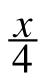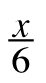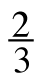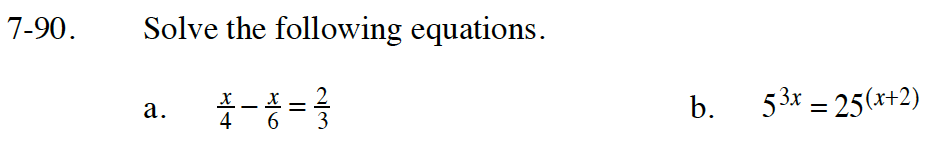Home > CC4 > Chapter 7 > Lesson 7.2.1 > Problem7-90

7-90.
1. Solve the following equations. Homework Help ✎

2.  a.–=b. 53x = 25(x+2)Read Math Note box for 3.3.2 to learn about fraction busters. What is the common multiple for 4, 6, and 3?

Look at problem 3-132, to review how to solve equations with exponents.

Can 25 be written with a base of 5?